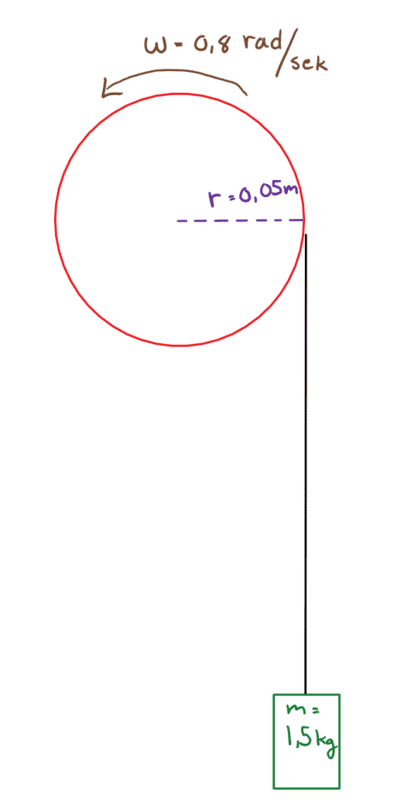Measure the torque of a servo motor

I have a servo motor which rotates 180 degrees. I want to calculate torque of the motor. To calculate the torque I'm thinking of lifting a load of a known value, with a known angular velocity. But I am struggling to find/calculate the torque based on these values. Does anyone know how to do this based on these known values?jrmichler
Mentor
If you are lifting the load at constant velocity, the torque is the force times the radius. Remember that force is in newtons and your weight is in kilograms.

In order to start the load moving, the load is accelerated. During the period of acceleration, the force is the weight of the mass plus mass times acceleration (F = ma).

Most servo motor drives can tell you the actual torque. They typically report torque as a percentage of the rated continuous torque. Most servo motors can deliver 3 or 4 times continuous torque for a short period of time. If the drive reports 250% torque, that is what is happening.

The motor is the motor, and the drive is the electronic box that sends power to the motor. There should be a data sheet that tells you the motor specifications, and also the performance of that motor with that drive.

If it is a stepping motor, it is not a servo motor. And servo motors are not stepping motors.

if you close the loop on a stepping motor, it becomes a servo motor. ;)